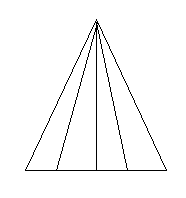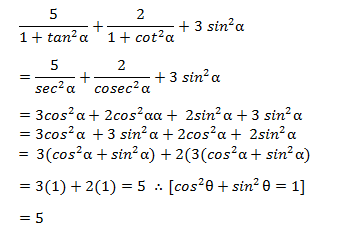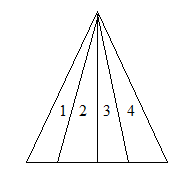# SSC CGL 2018 Practice Test Papers | Quantitative Aptitude (Day-20)

Dear Aspirants, Here we have given the Important SSC CGL Exam 2018 Practice Test Papers. Candidates those who are preparing for SSC CGL 2018 can practice these questions to get more confidence to Crack SSC CGL 2018 Examination.

[WpProQuiz 2957]

Click “Start Quiz” to attend these Questions and view Explanation

1) If A person travels with a speed of 60 kmph in certain distance, he will reach his destination in the estimated time. But He covered 2/3 of the distance in in 4/5 of estimated time.  Find the new speed by which he covers the remaining distance on time.

a) 100

b) 40

c) 80

d) 60

2) A and B starts a business in the ratio 4:5. A starts the business with an initial investment of Rs. 2500.After 3 years A withdraws Rs. 500 from his investment and B increases his investment by 500. Find the profit ratio after 5 years.

a) 61: 100

b) 133: 79

c) 92:133

d) 78: 151

3) A median bisects ________ of the triangle into two halves.

a) Area

b) Angle

c) Both angle and area

d) Either a or b

4) The sum of four positive numbers is odd. What is the minimum number of number of odd numbers to have sum of four numbers to be odd?

a) 1

b) 2

c) 3

d) 4

5) In how many ways a number 3780 are written as product of two different factors?

a) 20

b) 35

c) 30

d) 24

6) Find the value of 5/ (1+tan2α) +2/ (1+cot2α) +3 sin2α

a) 1

b) 6

c) 5

d) 2

7) Find how many triangles are there in the figure?a) 13

b) 11

c) 12

d) 10

8) If a/b= c/d then the value of (ab+cd)2 is equal to______

a) (a2+ c2) (b2+ d2)

b) a2b2+ c2d2

c) a2d2+ b2c2

d) (a2+ d2)(b2+c2)

9) A car normally covers a distance of 360 km at a speed of 45 km/hr. Due to some mechanical failure it halted in two places for different time periods in the ratio 3:5 and its average speed reduced to 30 km/hr. How long the car stopped at first place?

a) 1 hour

b) 3.5 hours

c) 2 hours

d) 1.5 hours

10)  The average of 15 consecutive natural numbers starting from 333 to 361 is x. Find the average if 4 more consecutive natural numbers are added before 333.

a) 347

b) 345

c) 343

d) 351

Let the estimated time= t, distance = x

S=60 kmph

S1=?, S2=?

X/t=60—-(i)

Sub (i) in (ii)

(2x/3)/(4t/5)= (10/12) (x/t)——–(ii)

S1=10/12 x60=50kmph

Remaining distance= x(1-2/3)= x/3

S2=(x/3)/(t/5)——(iii)

Sub (i) in (iii)

S2=5x/3t = 5/3* 60 =100 kmph

The new speed by which he covers the remaining distance on time = 100kmph

Investment of B= 2500/4×5=3125

Profit ratio of A: B = (2500×3) + (2000×2): (3125x 3)+ (3625×2)
= (7500+4000) : (9375+7250)
= 11500 : 16725

= 92:133

The line segment joining midpoint of a side to the vertex opposite to the side is called median. A median bisects area of the triangle intwo equal parts.

Let the four numbers be a, b, c and d respectively.

If all the 4 numbers are even, sum = even

If 1 number is odd and 3 numbers are even, then the sum = odd

Express the given number as prime factors.

3780= 22x 33x5x7

Total number of factors = (2+1) (3+1) (1+1) (1+1) = 3x4x2x2 = 48

No. of ways= 48/2=24Number of triangles= 1+2+3+4 = 10

Let a=c=2 and b=d=3

Substitute the value in (ab+cd)2

=144

Substitute the value in given options and find which of the equation value is equal to 144.

Option a) satisfies the condition

Original time = 360/45 = 8 hours

Time taken if the avg. speed is 30 km/hr = 360/30 = 12 hours

Car halted for 4 hrs in the ratio 3:5

Halted Time at the first place=   4/8*3=1.5 hrs